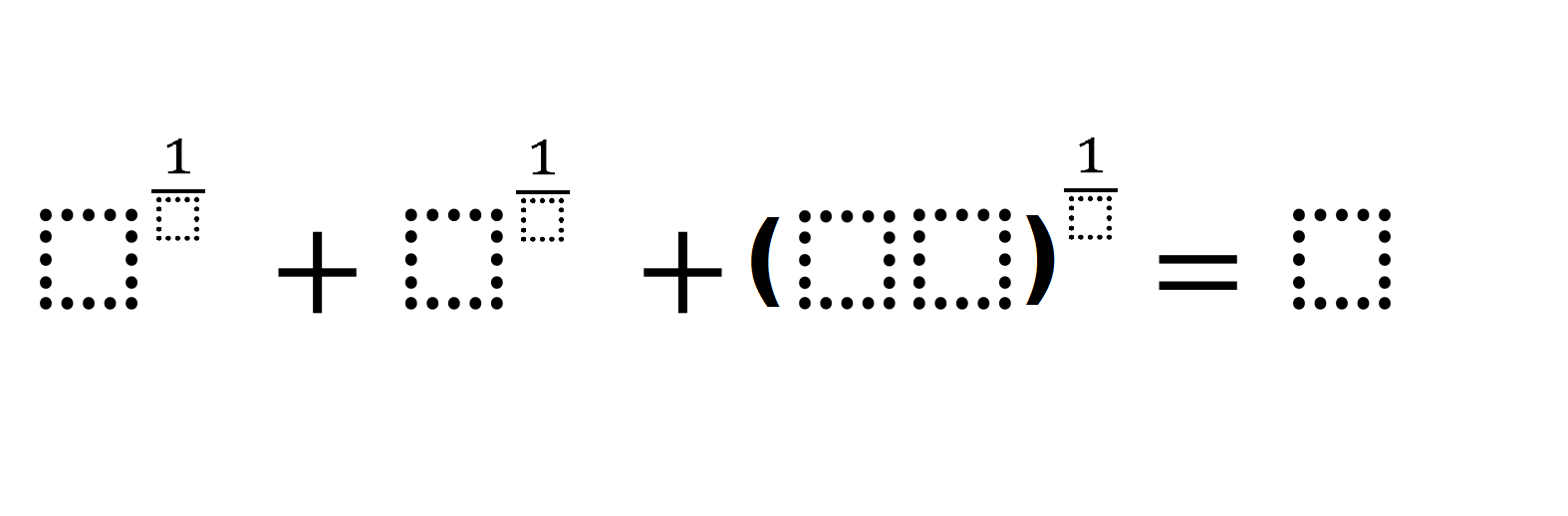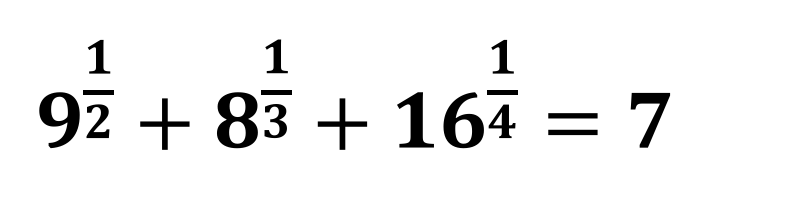# Fraction Exponents

Directions: Using the digits 1 to 9, at most one time each, fill in the boxes to make the statement true.### Hint

What do fraction exponents represent?

There are many possible answers, one is (see image):Source: Shaun Errichiello

## Imaginary Solutions to a Quadratic Equation

Directions: Using the digits 1 to 9 at most one time each, place a digit …

1.•